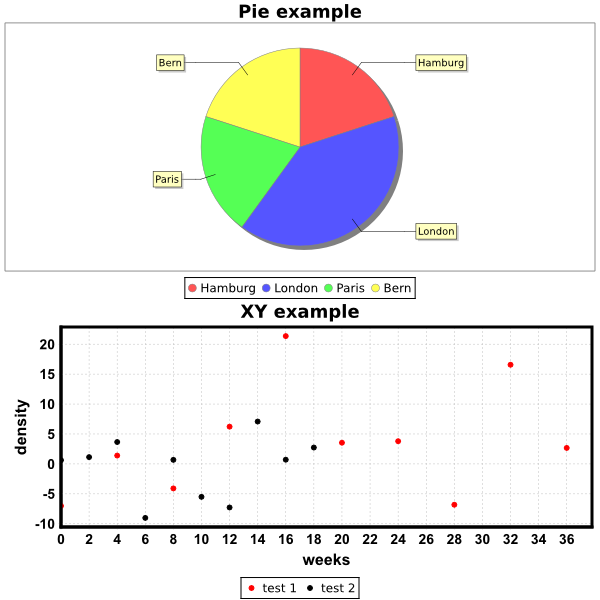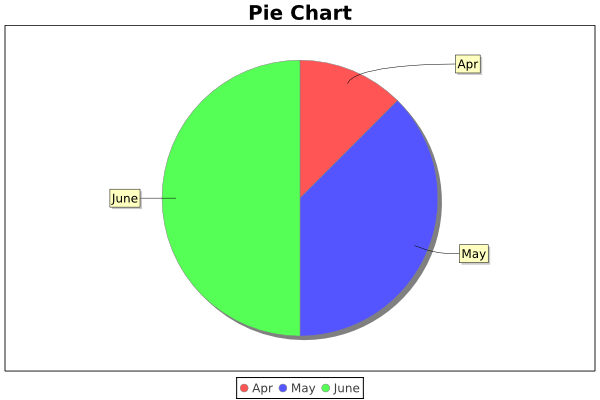# DMelt:Plots/7 Charts

Limitted access. First login to DataMelt if you are a full DataMelt member. Then login to HandWiki as a user.

## Charts

A chart is a graphical representation of data. Charts can be shown as bars (in a bar chart), lines (in a line chart), slices (in a pie chart) etc. Read the Chart article.

In DataMelt,jhplot.HChart canvas is used for showing charts. container. This canvas is important since it is the main canvas for the Android version of DataMelt and is based on JFreeChart. Here we will show a simple example based on Jython:

from java.awt import Color
from java.awt import Font
from java.util import Random
from math  import *
from jhplot import HChart,P1D

from java.lang import *

# test print streams using java
System.out.println("Test of OK message")
System.err.println("Test of Error message")

c1 = HChart("Canvas",600,600, 1, 2)
c1.setGTitle("Chart examples")
c1.cd(1,1)
c1.visible()

c1.cd(1,1)
c1.setChartPie()
c1.setName("Pie example")
c1.valuePie("Hamburg",1.0)
c1.valuePie("London",2.0)
c1.valuePie("Paris",1.0)
c1.valuePie("Bern",1.0)
c1.update()

# new plot
c1.cd(1,2)
c1.setName("XY example")
c1.setNameX("weeks")
c1.setNameY("density")
p1= P1D("test 1")
p1.setColor(Color.red)
p2= P1D("test 2")
# fill
rand = Random()
for i in range(10):
x=4.0*i # x-value
c1.update()

c1.export("a.eps")
# export to some image (png,eps,pdf,jpeg...)
# c1.export(Editor.DocMasterName()+".png")

The output shows pie charts and XY data as shown below:# Charts using jFreeChart

You can create plots using jFreeChart. One advantage of using jFreeChart is that it allows to build very custom plots (although less interactive than other canvases). When using with DataMelt, one can use the Dmelt methods for exporting JFreeChart images to EPS, PDF, SVG.0

Here is a small Jython/Python example that uses the jFreeChart API:

To learn about "chart" object, type "help.doc(chart")". The output of this code is a high-quality image :You can use jFreeChart for timeseries, charts, line plots, etc.